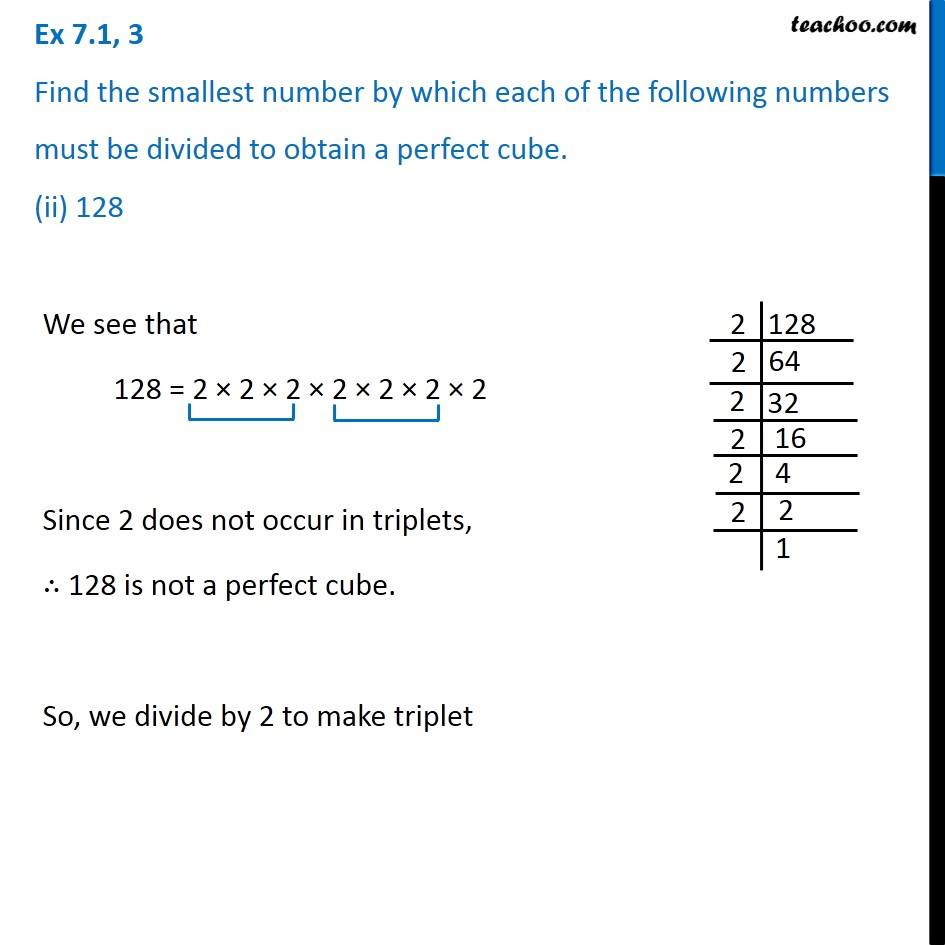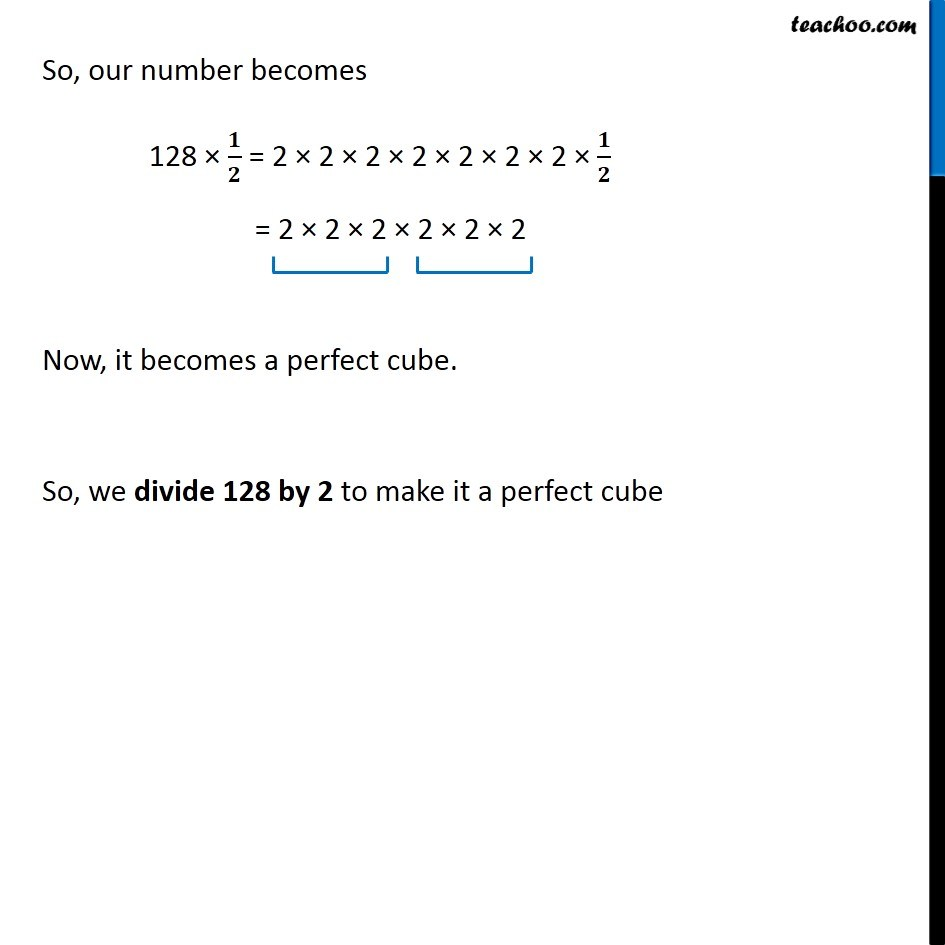Ex 6.1

Chapter 6 Class 8 Cubes and Cube Roots
Serial order wiseLearn in your speed, with individual attention - Teachoo Maths 1-on-1 Class

### Transcript

Ex 6.1, 3 Find the smallest number by which each of the following numbers must be divided to obtain a perfect cube. (ii) 128We see that 128 = 2 × 2 × 2 × 2 × 2 × 2 × 2 Since 2 does not occur in triplets, ∴ 128 is not a perfect cube. So, we divide by 2 to make triplet So, our number becomes 128 × 𝟏/𝟐 = 2 × 2 × 2 × 2 × 2 × 2 × 2 × 𝟏/𝟐 = 2 × 2 × 2 × 2 × 2 × 2 Now, it becomes a perfect cube. So, we divide 128 by 2 to make it a perfect cube